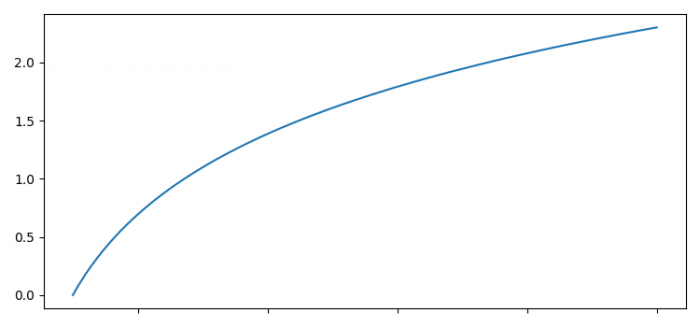# Hiding major tick labels while showing minor tick labels in Matplotlib

To hide major tick labels while showing minor ticklabels in Matplotlib, we can take the following steps −

• Set the figure size and adjust the padding between and around the subplots.

• Create x and y data points using numpy.

• Plot the x and y data points.

• Set a property on an artist object, using setp() method.

• To display the figure, use show() method.

## Example

import matplotlib.pyplot as plt
import numpy as np

plt.rcParams["figure.figsize"] = [7.50, 3.50]
plt.rcParams["figure.autolayout"] = True

x = np.linspace(1, 10, 100)
y = np.log(x)

plt.plot(x, y)

plt.setp(plt.gca().get_xmajorticklabels(), visible=False)

plt.show()

## OutputUpdated on: 10-Aug-2021

501 Views